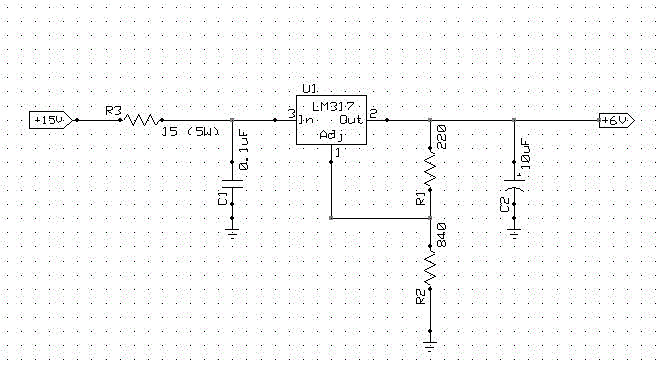Imming Home -> DC-DC Converter

# 15V DC to 6V DC Converter

Kerry Imming, kcimming@pobox.com, created 1/2/2000, last updated 02/23/2017.

Purpose: Convert 15V DC power output from a computer monitor to 6V DC to power computer speakers. This allows the computer speakers to power on/off automatically with the monitor.

The load placed on the 6V supply is approx. 40-250 mA. This was measured using a power supply and a set of Sony speakers.

The following circuit diagram shows a circuit that performs the voltage conversion using an LM317 adjustable regulator. Construction is not critical. I wired the circuit with point-to-point wiring on a perf board. Be sure to leave plenty of room for cooling for R3 and the heat sink on U1.The LM317 works by maintaining Vref = 1.25 V across V(out) and V(adj). I(adj), the current into the ADJ pin is approximately 50 uA and thus negligible. The value of Vout can be calculated as the voltage drop across R1 and R2. The voltage across R1 is known to be 1.25 volts (Vref). The current through R2 is assumed the same as that through R1 since Iadj is negligible. The relation of Vout to R1 and R2 can then be solved as follows:

Vout = IR2 * R2 + 1.25V

IR2 approx= IR1 = 1.25V / R1

Therefore: Vout = 1.25V + (1.25V / R1) * R2

or, Vout = 1.25V * (1 + R2 / R1)

R1 is picked arbitrarily. Let R1 = 220 ohms.

Solving for R2 gives:

R2 = (Vout / 1.25V - 1) * 220

Therefore, for Vout=6V, R2 = 836 ohms, which can be approximated by a series combination of 510 and 330 ohms.

R3 serves to dissipate some power to limit the power dissipated in the LM317. LM317 requires Vin > 7.5 volts to maintain Vout of 6 volts. Assuming a maximum current of 0.5 A:

R3 = V/I = (15V - 7.5 V) / 0.5A = 15 ohms MAX

### NOTES:

1. I used R3 =10 ohm, and C2 = 22 uF based on parts I had available.
2. R3 dissipates a significant amount of power (2-3 watts) and thus should be sized at at least 5 Watts.
3. LM317T should have a heat sink
4. Connectors for my monitor were 5.5mm O.D. and 2.1mm I.D. coaxial power plugs.
Monitor supply (15V) was positive center while speakers (6V) required a negative center.
Be sure to check required polarities and wire accordingly.
5. Another alternative is to connect the input to a +12 source obtained by connecting a "Y" splitter to a disk drive supply cable inside the computer. R3 would have to be < 9 ohms in this case.
6. LM337 corrected to LM317, 02/23/2017. Many thanks to Bob R. for pointing out this error.